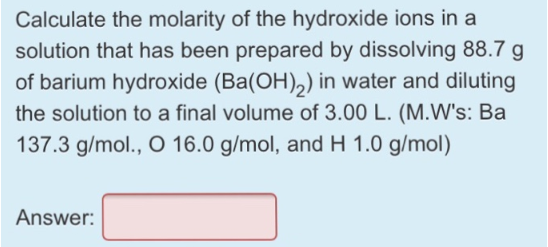# Problem: Calculate the molarity of the hydroxide ions in a solution that has been prepared by dissolving 88.7 g of barium hydroxide (Ba(OH)2) in water and diluting the solution to a final volume of 3.00 L. (M.W's: Ba = 137.3 g/mol, O = 16.0  g/mol, and H = 1.0 g/mol)

###### FREE Expert Solution
83% (119 ratings)###### Problem Details

Calculate the molarity of the hydroxide ions in a solution that has been prepared by dissolving 88.7 g of barium hydroxide (Ba(OH)2) in water and diluting the solution to a final volume of 3.00 L. (M.W's: Ba = 137.3 g/mol, O = 16.0  g/mol, and H = 1.0 g/mol)